CiteScore 5.2
More on impact ›

# Frontiers in Astronomyand Space Sciences## ORIGINAL RESEARCH article

Front. Astron. Space Sci., 12 May 2021 | https://doi.org/10.3389/fspas.2021.664483

# Exploring Three Recurrent Neural Network Architectures for Geomagnetic PredictionsPeter Wintoft* andMagnus Wik
• Swedish Institute of Space Physics, Lund, Sweden

Three different recurrent neural network (RNN) architectures are studied for the prediction of geomagnetic activity. The RNNs studied are the Elman, gated recurrent unit (GRU), and long short-term memory (LSTM). The RNNs take solar wind data as inputs to predict the Dst index. The Dst index summarizes complex geomagnetic processes into a single time series. The models are trained and tested using five-fold cross-validation based on the hourly resolution OMNI dataset using data from the years 1995–2015. The inputs are solar wind plasma (particle density and speed), vector magnetic fields, time of year, and time of day. The RNNs are regularized using early stopping and dropout. We find that both the gated recurrent unit and long short-term memory models perform better than the Elman model; however, we see no significant difference in performance between GRU and LSTM. RNNs with dropout require more weights to reach the same validation error as networks without dropout. However, the gap between training error and validation error becomes smaller when dropout is applied, reducing over-fitting and improving generalization. Another advantage in using dropout is that it can be applied during prediction to provide confidence limits on the predictions. The confidence limits increase with increasing Dst magnitude: a consequence of the less populated input-target space for events with large Dst values, thereby increasing the uncertainty in the estimates. The best RNNs have test set RMSE of 8.8 nT, bias close to zero, and linear correlation of 0.90.

## 1 Introduction

In this work we explore recurrent neural networks (RNNs) for the prediction of geomagnetic activity using solar wind data. An RNN can learn input–output mappings that are temporally correlated. Many solar terrestrial relations exhibit such behavior that contains both directly driven processes and dynamic processes that depend on time. The geomagnetic Dst index has been addressed in numerous studies and serves as a parameter for general space weather summary and space weather models. The Dst index is derived from magnetic field measurements at four near-equatorial stations and primarily indicates the strength of the equatorial ring current and the magnetopause current (Mayaud, 1980). The Dst index has attained a lot of attention over the years, both for understanding solar terrestrial relations and for use in space weather.

An early attempt to predict the Dst index from the solar wind made use of a linear filter (Burton et al., 1975) derived from the differential equation containing a source term (the solar wind driver) and a decay term. After removing the variation in Dst that is controlled by the solar wind dynamic pressure, one arrives at the pressure-corrected $Dst∗$ index (O’Brien and McPherron, 2000) which is modeled as

$dDst∗dt=Q−Dst∗τ,(1)$

where Q is the source term that depends on the solar wind and t is the decay time of the ring current. The decay time t may be a constant, but it may also vary with the solar wind. (see, e.g., the AK1 (constant τ) and AK2 (variable τ) models in O’Brien and McPherron (2000)). As the functional form of Q is not known, the equation is numerically solved by

$Dst∗(t+Δt)=Dst∗(t)+(Q(t)−Dst∗(t)τ(t))Δt.(2)$

Based on observed solar wind data, for hourly sampled data, the time step is $Δt=1$ hour. The source term Q is a nonlinear function of the solar wind parameters, and different forms have been suggested. The AK1 model defines the source term as

$Q(V,Bs)=aVBs nT/h,(3)$

where $a=−2.47$ is a constant, V is the solar wind speed (km/s), and $Bs$ is

$Bs={0,Bz≥0−Bz,Bz<0 nT.(4)$

$Bz$ is the vertical solar wind magnetic field component. Thus, as long as $Bz<0$, the Dst index will be driven to increasing negative values; for example, if τ is a constant and the solar wind conditions are constant with negative $Bz$, then $Dst∗$ will asymptotically approach $Q⋅τ$. With $τ=17$ hours (AK1), $V=600 km/s$ and $Bz=−20 nT$ give $VBs=12 mV/m$ and $Q⋅τ=−500$ nT. The AK1 model has been further extended by letting τ be a function of Dst and adding components for the diurnal and seasonal variation that are present in Dst (O’Brien and McPherron, 2002).

The machine learning (ML) approach could be viewed as a set of more general algorithms that can model complex functions. The development of an ML model is more involved and time consuming. For the prediction of the Dst index, many ML methods have been applied, and we here list some examples using different approaches: neural network with input time delays (Lundstedt and Wintoft, 1994; Gleisner et al., 1996; Watanabe et al., 2002), recurrent neural network (Wu and Lundstedt, 1997; Lundstedt et al., 2002; Pallocchia et al., 2006; Gruet et al., 2018), ARMA (Vassiliadis et al., 1999), and NARMAX (Boaghe et al., 2001; Boynton et al., 2011).

An RNN models dynamical behavior through internal states so that the output depends on both the inputs and the internal state (see, e.g., Goodfellow et al. (2016)) for an overview. Thus, structures that are temporally correlated can be modeled without explicitly parameterizing the temporal dependence; instead, the weights in the hidden layer that connects to the internal state units are adjusted during the training phase. An early RNN was the Elman network (Elman, 1990) which was applied to geomagnetic predictions (Wu and Lundstedt, 1997) and later implemented for real-time operation (Lundstedt et al., 2002). The Elman RNN can model complex dynamical behavior; however, it was realized that it could be difficult to learn dynamics for systems with long-range memory (Bengio et al., 1994). To overcome that limitation, other RNN architectures were suggested, such as the GRU (Cho et al., 2014) and LSTM (Hochreiter and Schmidhuber, 1997). The LSTM has been applied to geomagnetic predictions of the Kp (Tan et al., 2018) and Dst indices (Gruet et al., 2018; Xu et al., 2020). It should be noted that Elman RNN is less complex and has the shortest training times of the three architectures and may be suited for certain problems, and that it is not clear whether there is a general advantage of using GRU or LSTM (Chung et al., 2014; Goodfellow et al., 2016).

In this work, the main goal is to compare the three RNNs: Elman, GRU, and LSTM. The geomagnetic Dst index is chosen as target as it captures several interesting features of the geomagnetic storm with different temporal dynamics. The initial phase is marked by an increase in Dst caused by a directly driven pressure increase in the solar wind; the main phase is marked by a sudden decrease in Dst when solar wind energy enters the magnetosphere through mainly reconnection with southward $Bz$, and later, the storm enters the recovery phase when energy is dissipated by internal processes not related to the solar wind condition.

The inputs to the RNNs are solar wind, local time, and time of year. Specifically, past values of Dst are not used as inputs, although the autocorrelation is very strong (0.98). Clearly, all statistical measures of performance will improve for short lead time predictions when past values of Dst are used. However, as the solar wind controls the initial and main phases of the storm, the strong autocorrelation is mainly a result of quiet time variation and the relatively slow increase in Dst during the recovery phase. Another aspect is that for real-time predictions, the variable lead time given by the solar wind must be matched against available real-time Dst if it is used as input. Also, any errors in real-time Dst will affect the predictions, and as an example, during the period June–September 2020, the real-time Dst was offset by about $−30 nT$. It is also interesting to note that in a recent Dst prediction competition1 hosted by NOAA, it was stated that the models “may not take Dst as an input.”

As the idea here is to compare three RNN architectures that map from solar wind to Dst, the prediction lead time is not explored. The solar wind data used have been propagated to a location close to Earth, and no further lead time is added; thus, propagated solar wind at time t is mapped to $Dst(t)$. Clearly, possibilities to increase the lead time are of great interest, and many attempts have been made with models driven by measured solar wind (e.g., Gruet et al. (2018); Xu et al. (2020)). However, without any information other than L1 solar wind measurements, the initial phase cannot be predicted with any additional lead time, except that given by the L1-Earth solar wind propagation time, while the main phase may be predicted with possibly up to an additional hour due to magnetospheric processes. The effect of forcing models driven by measured solar wind to predict Kp and Dst with different lead times was studied in Wintoft and Wik (2018).

## 2 Models and Analysis

### 2.1 Models

A neural network performs a sequence of transforms by multiplying its inputs with a set of coefficients (weights) and applying nonlinear functions to provide the output. It has been shown that the neural network can approximate any continuous function (Cybenko, 1989). For a supervised network, the weights are adjusted to produce a desired output, given the inputs, known as the training phase. The training phase requires known input and target values, a cost function, and an optimization algorithm that minimizes the cost.

The Elman RNN was first developed for Dst predictions by Wu and Lundstedt (1997) and later implemented for real-time operation (Lundstedt et al., 2002). In this work, we use the term Elman network, but it is the same as the simple RNN used in the Tensorflow package that we use (Abadi et al., 2015). Using linear units at the output layer, the Elman network at time t is described by

$yt=b+∑j=1Jvjhtj=Vht,(5)$
$htj=f(aj+∑i=1Iwjixti+∑k=1Jujkht−1k)=f(Wxt+Uht−1)j,(6)$

with the output layer bias b, J hidden weights $vj$, nonlinear activation function f, J hidden layer biases $aj$, $J×I$ weights $wji$, and $J×J$ recurrent weights $ujk$. Note that we use superscripts as indices, not powers. The equations can be written more condensed using weight matrices $W$ and $U$, where the bias terms $(aj,b)$ have been collected into the matrices and increasing the lengths of $xt$ and $ht$ by adding a constant set to one. For example, in Lundstedt et al. (2002), there are $I=3$ inputs $(Bz,n,V)$ and $J=4$ hidden units.

A minimalistic Elman network can be constructed by using only one input unit and one linear unit in the hidden layer, thus $b=0$, $v1=1$, leading to

$yt=a1+w11xt1+u11yt−1,(7)$

which after some rearranging can be written as

$yt=yt−1+a1+w11xt1−(1−u11)yt−1,(8)$

which is identical to Eq. 2 for $τ=const$ and $Δt=1$, and by letting $a1=0$, $w11xt1=Q(t)$, and $1−u11=1/τ$. This simple network is trained using the pressure-corrected Dst index as the target. As the weights in the network are initiated with random values before training begins, there will be some variation in the final weight values if the training is repeated. We find typical values of $w11$ and $u11$ corresponding to $a∈[−2.4,−2.7]$ (Eq. 3) and $τ∈[14,16]$ hours, which are close to the values used by O’Brien and McPherron (2000). However, the algorithm can get stuck in local minima that results in quite different values. We provide code on Github2 for the minimalistic Elman network (see Model005.py).

The gated recurrent unit (GRU) neural network (Cho et al., 2014) has a more complex architecture than the Elman network. We implement a single GRU layer, and the output from the network is as before, given by $yt=Vht$ (Eq. 5). The GRU layer output at unit j is

$htj=ztjht−1j+(1−ztj)h˜tj,(9)$

where $ztj$ is the update gate and $h˜tj$ is the candidate activation. The update gate is defined as

$ztj=σ(Wzxt+Uzht−1)j,(10)$

where σ is the sigmoid function with output range 0–1. The weight matrix $Wz$ operates on the input vector $xt$, and the matrix $Uz$ operates on the past activation $ht−1$. The candidate activation is defined as

$h˜tj=f(Wxt+U(rt⊙ht−1))j,(11)$

where f is a nonlinear function with two additional weight matrices $W$ and $U$. The $U$ matrix operates on the past activation weighted by the reset gate

$rtj=σ(Wrxt+Urht−1)j,(12)$

which has a further set of weights matrices $Wr$ and $Ur$. Clearly, the GRU network is more complex than the Elman network, and it has approximately 3 times more weights than the Elman network for the same number of units. As the update and reset gates have outputs between 0 and 1, we see that when both produce ones $[(ztj,rtj)=(1,1)]$, the GRU network simplifies to the Elman network. On the other hand, when $ztj=0$, no information of the current input $xt$ is used, only the past state $ht−1$. Finally, when $rtj=0$, no information of past states goes through the candidate activation (Eq. 11); information on past states only goes through Eq. 9 and is weighted by $1−ztj$.

The long short-term memory (LSTM) neural network (Hochreiter and Schmidhuber, 1997) was introduced before GRU and has further complexity with the number of weights approximately four times that of the Elman network, given the same number of units. We will not repeat the equations here but instead refer to for example, Chung et al. (2014). The LSTM has three gating functions, instead of GRU’s two, that control the flow of information: the output gate, the forget gate, and the input gate. When they have values 1, 0, and 1, respectively, the LSTM simplifies to the Elman network.

Given a network with a sufficient number of weights, it can be trained to reach zero MSE; however, such a network will have poor generalizing capabilities; that is, it will have large errors on predictions on samples not included in the training data. Different strategies exist to prevent over-fitting (Goodfellow et al., 2016). We apply early stopping and dropout (Srivastava et al., 2014; Gal and Ghahramani, 2016).

In order to make a robust estimation of the performance of the networks, we apply k-fold cross-validation (Goodfellow et al., 2016). During a training session, one subset is held out for testing and the remaining $k−1$ subsets are used for training and validation, and out of the $k−1$ subsets, one is used for validation and the remaining $k−2$ subsets for the training. During training, the validation mean squared error (MSE) is monitored, and the network with lowest validation MSE is chosen (early stopping). In practice, to know that the minimum validation MSE has been reached, the training is continued for a number of epochs after the lowest MSE has been reached. The final evaluation of the models is performed on the k different test sets (see Section 2.2 to know how the different sets are selected).

### 2.2 Data Sets

The hourly solar wind data and Dst index are obtained from the OMNI dataset (King and Papitashvili, 2005). The inputs are the solar wind magnetic field magnitude B, the y- and z-components $(By,Bz)$ in the geocentric solar magnetospheric (GSM) coordinate system, the particle density n, and speed V. To provide information on diurnal and seasonal variations (O’Brien and McPherron, 2002), four additional variables are added: the day of year parameterized as sine and cosine of $2πDOY/365$, and local time as sine and cosine of $2πUT/24$. Thus, in total, nine input variables. Several previous geomagnetic prediction models also use diurnal and seasonal inputs (e.g., Temerin and Li (2006); Wintoft et al. (2017); Wintoft and Wik, (2018)). Many different coupling functions (Q) for the dayside reconnection rate have been suggested and investigated (Borovsky and Birn, 2014), but as the neural network can approximate any function, the exact function does not have to be specified as long as the relevant inputs are available.

The target variable (Dst) depends on both the solar wind and past states of the system, where past states can be described by past values of Dst itself or by past values of the solar wind. We choose to only include solar wind, thereby not relying on past observed or predicted values of Dst. For the RNN training algorithm, the data are organized so that the past T solar wind observations are presented at each time step. The input data are thus collected into a $N×T×9$ tensor, and the target data have $N×1$ dimension, where N is the number of samples in the set. The input history should be long enough to capture typical storm dynamics, and we found that validation errors leveled out at $T=120$ hours (see also the results regarding T in Sections 2.3 and 2.4).

To implement the k-fold cross-validation (CV), the dataset must be partitioned into subsets; we perform a five-fold CV. We choose the five sets to each have similar target (Dst) mean and standard deviation so that training, validation, and testing are based on comparable data. If a more blind approach were done, then there is a high risk that training is performed on data dominated by storms, while testing is performed on more quiet conditions. Further, the samples in a subset cannot be randomly selected because there will be considerable temporal overlap between samples of different subsets due to the $T=120$ hour window. Instead, we build the subsets from data covering complete years. The data we use cover the years 1995 to 2015, extending over almost two solar cycles and with few solar wind data gaps. We define five subsets based on the data for the years shown in Table 1. The datasets used for training, validation, and test are selected by cycling through the subsets. For the first CV (CV-1) subset, one is selected as test set; subsets two, four, and five for training; and subset three for validation. The process is repeated according to Table 2.

TABLE 1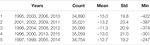TABLE 1. Summary of the five subsets showing the years, number of samples, the mean (nT), standard deviation (nT), and minimum Dst (nT).

TABLE 2

The input and target values span very different numerical ranges, whereas the training algorithm should receive input-target data that have similar numerical ranges. Therefore, the input and target data are normalized, where the normalization coefficients are found from the training set. By subtracting the mean and dividing with the standard deviation for each variable separately, the training set will have zero mean and one standard deviation on all its inputs and target variables. However, as the distributions for each variable are highly skewed, they result in several normalized values with magnitudes much larger than one. Another way to normalize is to instead rescale the minimum and maximum values to the range [-1, 1]. This guarantees that there will be no values outside this range for the training set. We found that the min–max normalization gave slightly better results, especially at the large values.

### 2.3 Hyperparameters

There are a number of hyperparameters (HPs) that control the model complexity and training algorithm that need to be tuned, but it is not feasible to make an exhaustive search. Initially, a number of different combinations of HP values were manually tested to provide a basic insight into reasonable choices and how the training and validation MSEs vary with epochs. In this initial exploration, we found the Tensorboard (Abadi et al., 2015) software valuable in monitoring the MSE.

The Adam learning algorithm (Kingma and Lei, 2015), which is a stochastic gradient descent method, has three parameters: learning rate ϵ and two decay rates for the moment estimates $(β1,β2)$. We fix the latter to the suggested values $β1,β2=0.9,0.999$ and vary $ε∈[5⋅10−4,1⋅10−3,2⋅10−3]$.

The learning algorithm updates the weights in batches of samples from the training set, where the number of samples in each batch $NB$ is much smaller than the total number of training samples $(NB≪N)$. We test batch sizes of $NB∈[32,64,128]$. One training epoch includes approximately $N/NB$ training iterations in which the weights are updated at each iteration.

The model capacity is determined by the number of weights and the network architecture. In this work, we have one input layer, a recurrent layer (hidden layer), and a single output. Thus, the capacity is determined by the network type $(M∈[Elman,GRU,LSTM])$ and the number of hidden units $(NH)$.

The current state $(ht)$ in the RNN depends on both its inputs $(xt)$ and the past state $(ht−1)$. For computational performance reasons, past states are not kept indefinitely; instead, there is a limit T on the length of the memory. We explored $T∈[48,72,96,120]$ hours and found that the validation MSE decreased with increasing T, but that it leveled out for large T. We therefore set $T=120$ hours. This also means that any dynamical processes extending past 120 h cannot be modeled internally by the RNN. The choice of T for the Elman and GRU networks is studied on simulated Dst data in the next section.

The dropout is controlled by parameters that specify the fraction of network units in a layer that are randomly selected per epoch and temporarily disregarded. The dropout can be applied to all layers: the input layer $(di)$, the recurrent layer $(dr)$, and the hidden layer $(dh)$. The dropout is a number between 0 and 1, where 0 means all units are included and 1 that all units are unused.

For each combination of HP that we explore, we train three networks initiated with different random weight values as there is no guarantee that the training algorithm will find a good local minimum. The network with the lowest validation error is selected. Note that here validation refers to the split into training and validation sets used during training, which is different from the cross-validation sets that make up the independent test set.

### 2.4 Training Network on Simulated Data

It is interesting to study the RNNs on data generated from a known function relating solar wind to Dst, and for this purpose, we use the AK1 model (O’Brien and McPherron, 2000). Using the datasets defined in the previous section, we apply the AK1 model to the solar wind inputs and create the target data. Thus, there exists an exact relation between input and output, and the learning process of the RNN will only be limited by the amount of data, network structure (type of RNNs), and network capacity (size of RNNs). We showed that the minimalistic Elman network (Eq. 7) can model the pressure-corrected Dst. The AK1 model also includes the pressure term, and its inputs are $Bz$, n, and V. The five-fold CV is applied to Elman and GRU networks, and we vary the time window T and the network size $NH$.

In Figure 1, the validation errors as function of T are shown for the Elman and GRU networks. At each T, the optimal networks with respect to $NH$ are used. We see that for small T, the RMSE is large, but it is similar for the two network types. At small T, only part of the storm recovery phase can be modeled. But as T is increased, the RMSE becomes much smaller for the GRU network than for the Elman network. It is likely that the Elman network suffers from the vanishing gradient problem (Bengio et al., 1994): the reason for introducing GRU and LSTM networks. We also see that the GRU network reaches an RMSE of lower than 0.6 nT, which could be further decreased by increasing T. Thus, the GRU network can learn the AK1 model using the observed solar wind data.

FIGURE 1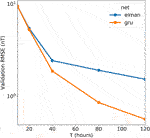FIGURE 1. The average validation RMSE as function of memory size (T in hours) for the Elman and GRU networks.

### 2.5 Result for the Dst Index

As described in Section 2.2, the inputs to the Dst model are solar wind magnetic field $(B,By,Bz)$, density (n), and speed (V); the day of year parameterized as sine and cosine of $2πDOY/365$; and local time as sine and cosine of $2πUT/24$. The DOY and UT are added to model the seasonal and diurnal variations in Dst (O’Brien and McPherron, 2002).

We perform a search in the hyperparameter space as described above and conclude that training is not very sensitive on the learning rate (ϵ) or batch size $(NB)$, and therefore fix them at $(ε,NB)=(10−3,128)$.

For each of the five splits, we select the corresponding training set (Tables 1,2), and RNNs are trained with different number of hidden units $(NH)$ and different dropout rates $(di,dr,dh)$. For each combination of $(NH,di,dr,dh)$, three networks are trained starting from different random initial weights. During training, the validation error is monitored, and the network with lowest validation error is selected. The training is stopped 20 epochs after the minimum validation error has been reached, and the network at minimum validation error is saved. Typically, the minimum validation RMSE is found after 40 to 80 epochs. This results in five different networks for each HP combination that can be tested using the CV approach. See Appendix for software used and typical training times.

The coupling function from solar wind to observed Dst is subject to a number of uncertainties, and to provide a few examples: the solar wind data have been measured at different locations upstream of Earth, mostly from orbit around the L1 location, and then shifted to a common location closer to Earth (King and Papitashvili, 2005); We rely on a point measurement; there may be both systematic and random errors in the derived Dst index. The uncertainties introduce errors in the input–output mapping, and to reduce their effect and improve generalization, we apply dropout. From a search of different combinations of $(NH,di,dr,dh)$, it was found that dropout on the inputs $(di)$ always led to poor performance, which can be understood as several inputs individually are critical, for example $Bz$. Therefore, we set $di=0$. The performance improved when dropout was applied on the recurrent and hidden layers. Figure 2 shows the training and validation RMSE as a function of $NH$ for different dropouts. In the case with no dropout (left panel), it is seen that the GRU and LSTM validation errors are similar and significantly below the Elman validation errors. There is also a large gap between the training and validation errors, indicating over-fitting on the training set. When dropout is introduced (middle and right panels), the network size must be increased to reach similar validation RMSE as when no dropout is applied, which is expected as only a fraction of units are active at any one time. But we also see that the gap between training and validation errors decreases. We also applied dropout on the Elman network, but the validation errors became large when $dr>0$; therefore, the results are not included in the middle and right panels. When $dr=0.5$ and $dh=0.5$ (right panel), the optimal GRU and LSTM networks have $NH=50$ and $NH=40$, respectively. In terms of the number of weights, they are of similar sizes, 9,051 and 8,041, respectively. When dropout is applied, the number of active weights drops to 2,651 and 2,421

FIGURE 2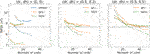FIGURE 2. The average training (dashed lines) and validation (solid lines) RMSE as function of the number of hidden units ($NH$) for the Elman, GRU, and LSTM networks. The panels show errors, from left to right, when no dropout is applied ($dr=0,dh=0$), dropout $dr=0.5,dh=0.3$, and dropout $dr=0.5,dh=0.5$. Note that the dropout on the inputs is $di=0$. Gray horizontal line marks the minimum validation RMSE.

For each CV set, we select the GRU and LSTM networks with minimum validation RMSE with and without dropout, and run them and collect the 5 CV sets into one set. We thereby get an estimate of the generalization performance for the whole 1995 to 2015 period. Figure 3 shows scatterplots of predicted Dst vs. observed Dst on the test sets for different networks. Table 3 summarizes the performance on the training, validation, and test sets. The 95% confidence intervals have been estimated by both assuming independent data points and taking into account the autocorrelation (Zwiers and von Storch, 1995). It is clear that using dropout significantly improves the generalization capability. We also see that there is no significant difference between the GRU and LSTM networks. The bias (mean of errors) and linear correlation coefficient are computed on the test set and shown in Table 4.

FIGURE 3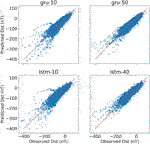FIGURE 3. Scatterplots of predicted vs. observed Dst based on the five CV test sets. The left panels show predictions without dropout using GRU and LSTM networks (gru-10 and lstm-10), while the right panels are predictions based on networks trained using dropout of $(dr,dh)=(0.5,0.5)$ (gru-50 and lstm-40).

TABLE 3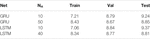TABLE 3. Training, validation, and test RMSE (nT) for networks with and without dropout. The training and validation RMSE are averages over the five CV splits, while the test RMSE is computed from the combined five CV test sets. Networks with $NH=10$ have no dropout and the larger networks have dropout $(dr,dh)=(0.5,0.5)$. The 95% RMSE confidence interval is approximately $±0.03 nT$ assuming independent errors but increases to $±0.17 nT$ if the autocorrelation is taken into account.

TABLE 4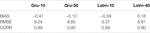TABLE 4. BIAS, RMSE, and CORR for the GRU and LSTM models on the test set. BIAS and RMSE are in units of nT. The 95% confidence intervals are $±0.04$ to $±0.2 nT$ for BIAS, $±0.03$ to $±0.17 nT$ for RMSE (same as Table 3), and $±0.001$ to $±0.01$ for CORR. The lower limits assume independence and the higher limits take into account the autocorrelations.

The performance of the networks varies with the level of Dst; the errors have a tendency to increase with the magnitude of Dst. Figure 4 shows the RMSE binned by observed Dst. Down to $Dst=−300 nT$, the networks with dropout have the lowest RMSE. The bin at $Dst=−400 nT$ has too few samples to be interpretable. The main reason the errors increase with the magnitude of Dst is that there are very few samples around the extremes; thus, the uncertainty of the function estimation will be large. In Wintoft et al. (2017), this problem was addressed by using an ensemble of networks; the predictions from several networks with different weights were averaged. In this work, we study the use of dropout not only in the training phase but also in the prediction phase. The algorithm that temporarily cancels units at random during training can also be applied during prediction. This means that there is practically an indefinitely number of weight combinations that can be used to produce an arbitrary number of predictions at each time step. For the GRU network with $(NH,dr,dh)=(50,0.5,0.5)$, there are more than $1028$ possible combinations. There is a Bayesian interpretation of dropout as the estimation of model uncertainty (Gal and Ghahramani, 2016b). The idea is that the weights are random variables leading to a distribution of predictions for fixed inputs. For each sample, a large number of predictions can be generated, which randomly use different combinations of network units. For each sample, we generate 100 predictions and compute the mean and standard deviation. Figure 5 shows two examples, the first a severe geomagnetic storm and the second a major storm. The mean predictions with dropout come close to the predictions without dropout. The prediction uncertainty is small during quiet times (Dst close to zero) and increases with storm magnitude. Again, this is a result of the greater uncertainty in parameter estimates in regions which are poorly sampled.

FIGURE 4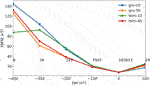FIGURE 4. The test RMSE binned by observed Dst. The RMSE is computed on the five CV test sets. Bins are 100 nT wide, and the numbers show the number of samples in each bin. Legend: GRU (gru-10) and LSTM (lstm-10) networks without dropout, and GRU (gru-50) and LSTM (lstm-40) networks with dropout $dr=0.5,dh=0.5$.

FIGURE 5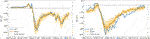FIGURE 5. Two geomagnetic storms predicted with the GRU network using the test set. Panels show observed Dst (blue solid), predicted Dst without dropout during prediction phase (dashed green), and mean prediction with dropout (orange solid). The dark orange regions show the predicted $±σ$ and the light orange regions the $±2σ$. The dash-dotted curve is the quiet time variation. Note that the intensity of the storms is different and that the y-scales span different ranges.

As time of day and season are included in the inputs, the network can model diurnal and seasonal variations in Dst. These variations are not strong, and the left panel in Figure 6 shows Dst for all years averaged over month and UT hour. Running the GRU networks on the test data from the five CV sets reveals a very similar pattern (second panel from left). Thus, the network shows similar long-term statistics considering that it is driven only by solar wind and time information. The two left panels contain contributions from all levels of Dst from quiet conditions to storm conditions. But we may now simulate solar wind conditions that we can define as quiet conditions. The two right panels show predicted Dst, assuming solar wind flowing out from the Sun (GSEQ system) along the Parker spiral with a $45∘$ angle at L1 at two different speeds, 350 km/s and 400 km/s, respectively. In this configuration, $Bz=0$ in the solar coordinate system, but via geometric effects (Sun’s and Earth’s tilts with respect to the ecliptic and Earth’s dipole tilt), $Bz$ will be nonzero in the GSM system showing diurnal and seasonal variations (Lockwood et al., 2020).

FIGURE 6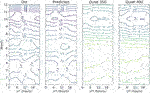FIGURE 6. The panels show average Dst binned by month and UT hour. From left to right, the averages are based on all observed Dst (Dst), predicted Dst from the CV test sets (Predicted), predicted Dst from quiet solar wind at 350 km/s (Quiet 350), and predicted Dst from quiet solar wind at 400 km/s (Quiet 400) (see text for definition of quiet solar wind).

## 3 Discussion and Conclusion

There is a close correspondence between Elman networks and models expressed in terms of the differential equation for the Dst index. A minimalistic Elman network trained on simulated data from the pressure-corrected Dst index (Eq. 1) results in weights that translate to values around $a=2.45$ and $τ=15$, close to those used in Eqs 2,3. However, using solar wind data from the years 1995–2015 and targeting simulated Dst from the AK1 model, we find that the RMSE for Elman network basically levels out for temporal history of $T≳40$ hours. This is not the case for the GRU network, which has similar RMSE up to $≲20$ hours but continues to improve for $T>20$ hours. We interpret this as an effect of the vanishing gradient problem (Bengio et al., 1994) that is solved in the GRU and LSTM networks. It should be noted that the Elman network takes less time to train, and if the dynamics of the system can be captured in less than about 20 time steps, then the Elman network could be sufficient. In the future, it would be interesting to perform similar experiments for other solar terrestrial variables, for example, other geomagnetic indices with different temporal dynamics. Another line of experimenting could be to separate processes with different dynamics in the construction of the RNN.

The GRU (Cho et al., 2014) and LSTM (Hochreiter and Schmidhuber, 1997) networks include gating units that control information flow through time. However, it is not clear if one architecture is better than the other (Chung et al., 2014). In order to reliably study the differences between the two RNNs, we applied five-fold cross-validation. Further, it was also essential to apply dropout (Gal and Ghahramani, 2016) to reduce over-fitting and achieve consistent results. Using solar wind data and observed Dst from 1995 to 2015, we see no significant difference between the two architectures. However, the GRU network is slightly less complex than the LSTM and will therefore have shorter training times.

An interesting effect of using dropout is that it can also be applied during the prediction phase as a way of capturing model uncertainty (Gal and Ghahramani, 2016b). Using dropout during prediction is similar to ensemble prediction based on a collection of networks with identical architectures but different specific weights (Wintoft et al., 2017), but with the great advantage that the predictions can be based on, in principle, unlimited number of models. However, it is different from using an ensemble of different types of models like in Xu et al. (2020). We illustrated the prediction uncertainty using dropout for a couple of storms from the test set. Estimating the prediction uncertainty is important and was addressed by Gruet et al. (2018) using a combination of LSTM network and a Gaussian process (GP) model. In that case, the LSTM network provides the mean function to the GP model from which a distribution of prediction can be made. For future work, it will be interesting to further study the use of dropout for estimating model uncertainty.

Predictions based on the test sets using the GRU networks show very good agreement with observed Dst when averaged over month and UT (Figure 6). The semiannual variation (Lockwood et al., 2020) is clear, with a deeper minimum in autumn than in spring and a weak UT variation. It is a combination of geometrical effects that cause the asymmetric semiannual variation leading to a modulation of the $Bz$ component in the GSM system, and, together with the nonlinear solar wind–magnetosphere coupling, gives rise to the variation in Dst. The two rightmost panels in Figure 6 show predictions based on simulated data with $Bz=0$ in the GSEQ system using two different speeds. In these cases, the semiannual variation is only caused by geometrical effects, while the two panels to the left also contain storms caused by different solar wind disturbances like coronal mass ejections. We also see that the difference between the spring and autumn minima is about 6 nT for both observed and predicted Dst, while the difference is about 14–18 nT for quiet time-simulated Dst. In this work, we only showed that the semiannual variation is reproduced by the simulations, but for the future, other types of simulations that contain CME structures could be performed to provide further insights into the semiannual variations.

## Data Availability Statement

Publicly available datasets were analyzed in this study. These data can be found here: https://omniweb.gsfc.nasa.gov/ow.html.

## Author Contributions

PW and MW have carried out this work with main contribution from PW.

## Conflict of Interest

The authors declare that the research was conducted in the absence of any commercial or financial relationships that could be construed as a potential conflict of interest.

## Acknowledgments

We acknowledge the use of NASA/GSFC’s Space Physics Data Facility’s OMNIWeb (or CDAWeb or ftp) service and OMNI data.

## Appendix: software and hardware

The code has been written in Python where we rely on several software packages: Pandas for data analysis (T. pandas-dev/pandas: Pandas, 2020); Matplotlib for plotting (Hunter, 2007); and TensorFlow and TensorBoard for RNN training (Dataset] Abadi et al., 2015).

The simulations have been run on an Intel Core i9-7960X CPU at 4.2 GHz with 64 GB memory. In total, 32 threads can be run in parallel. Typical training time for one Elman network with 30 hidden units for 50 epochs ranges between 5 and 15 min, where the shorter time is due to that the process could be distributed on multiple threads. We noted that one training process could be distributed over four threads, when the overall load was low. A GRU network with 10 hidden units could take between 30 min and slightly more than 1 h for 50 epochs. A 10-hidden unit LSTM network ranged between 50 min and 1.5 h.

## References

Abadi, M., Agarwal, A., Barham, P., Brevdo, E., Chen, Z., Citro, C., et al. (2015). TensorFlow: Large-Scale Machine Learning on Heterogeneous Systems. Software available from tensorflow.org.

Bengio, Y., Simard, P., and Frasconi, P. (1994). Learning Long-Term Dependencies with Gradient Descent Is Difficult. IEEE Trans. Neural Netw. 5 157–166. doi:10.1109/72.279181

Boaghe, O. M., Balikhin, M. A., Billings, S. A., and Alleyne, H. (2001). Identification of Nonlinear Processes in the Magnetospheric Dynamics and Forecasting of Dst Index. J. Geophys. Res. 106, 30047–30066. doi:10.1029/2000ja900162

Borovsky, J. E., and Birn, J. (2014). The Solar Wind Electric Field Does Not Control the Dayside Reconnection Rate. J. Geophys. Res. Space Phys. 119 751–760. doi:10.1002/2013JA019193

Boynton, R. J., Balikhin, M. A., Billings, S. A., Sharma, A. S., and Amariutei, O. A. (2011). Data Derived Narmax Dst Model. Ann. Geophys. 29 965–971. doi:10.5194/angeo-29-965-2011

Burton, R. K., McPherron, R. L., and Russell, C. T. (1975). An Empirical Relationship between Interplanetary Conditions andDst. J. Geophys. Res. 80 4204–4214. doi:10.1029/ja080i031p04204

Cho, K., van Merriënboer, B., Gulcehre, C., Bahdanau, D., Bougares, F., Schwenk, H., et al. (2014). “Learning Phrase Representations Using RNN Encoder–Decoder for Statistical Machine Translation,”in Proceedings of the Conference on Empirical Methods in Natural Language Processing (EMNLP) (Doha, Qatar: Association for Computational Linguistics) 1724–1734. doi:10.3115/v1/D14-1179

Chung, J., Gulcehre, C., Cho, K., and Bengio, Y. (2014). Empirical Evaluation of Gated Recurrent Neural Networks on Sequence Modeling. NIPS 2014 Workshop on Deep Learning, December 2014.

Cybenko, G. (1989). Approximation by Superposition of a Sigmoidal Function. Math. Control Signals, Syst. 2 303–314. doi:10.1007/bf02551274

Elman, J. L. (1990). Finding Structure in Time. Cogn. Sci. 14 179–211. doi:10.1207/s15516709cog1402_1

Gal, Y., and Ghahramani, Z. (2016a). “A Theoretically Grounded Application of Dropout in Recurrent Neural Networks,” in 30th Conference on Neural Information Processing Systems (Barcelona, Spain: NIPS).

Gal, Y., and Ghahramani, Z. (2016b). Dropout as a Bayesian Approximation: Representing Model Uncertainty in Deep Learning. Proceedings of the 33rd International Conference on Machine Learning, New York, NY, United States, 2016, (JMLR: W&CP), 48.

Gleisner, H., Lundstedt, H., and Wintoft, P. (1996). Predicting Geomagnetic Storms from Solar-Wind Data Using Time-Delay Neural Networks. Ann. Geophys. 14 679–686. doi:10.1007/s00585-996-0679-1

Goodfellow, I., Bengio, Y., and Courville, A. (2016). Deep Learning (MIT Press)

Gruet, M. A., Chandorkar, M., Sicard, A., and Camporeale, E. (2018). Multiple-hour-ahead Forecast of the Dst Index Using a Combination of Long Short-Term Memory Neural Network and Gaussian Process. Space Weather 16, 1882, 1896. doi:10.1029/2018SW001898

Hochreiter, S., and Schmidhuber, J. (1997). Long Short-Term Memory. Neural Comput. 9 1735–1780. doi:10.1162/neco.1997.9.8.1735

Hunter, J. D. (2007). Matplotlib: A 2d Graphics Environment. Comput. Sci. Eng. 9 90–95. doi:10.1109/MCSE.2007.55

King, J. H., and Papitashvili, N. E. (2005). Solar Wind Spatial Scales in and Comparisons of Hourly Wind and Ace Plasma and Magnetic Field Data. J. Geophys. Res. 110, A02104. doi:10.1029/2004JA010649

Kingma, D. P., and Lei, Ba. J. (2015). “Adam: A Method for Stochastic Optimization,” in The 3rd International Conference on Learning Representations (ICLR), arXiv:1412.6980.

Lockwood, M., Owens, M. J., Barnard, L. A., Haines, C., Scott, C. J., McWilliams, K. A., et al. (2020). Semi-annual, Annual and Universal Time Variations in the Magnetosphere and in Geomagnetic Activity: 1. Geomagnetic Data. J. Space Weather Space Clim. 10 . doi:10.1051/swsc/2020023

Lundstedt, H., Gleisner, H., and Wintoft, P. (2002). Operational Forecasts of the geomagneticDstindex. Geophys. Res. Lett. 29, 34. doi:10.1029/2002GL016151

Lundstedt, H., and Wintoft, P. (1994). Prediction of Geomagnetic Storms from Solar Wind Data with the Use of a Neural Network. Ann. Geophys. 12, 19–24. doi:10.1007/s00585-994-0019-2

Mayaud, P. N. (1980). Derivation, Meaning, and Use of Geomagnetic Indices, Geophysical Monograph. 22 (American Geophysical Union.

O’Brien, T. P., and McPherron, R. L. (2000). Forecasting the Ring Current Dst in Real Time. J. Atmos. Solar-Terrestrial Phys. 62 1295–1299.

O’Brien, T. P., and McPherron, R. L. (2002). Seasonal and Diurnal Variation of Dst Dynamics. J. Geophys. Res. 107, 1341. doi:10.1029/2002JA009435

Pallocchia, G., Amata, E., Consolini, G., Marcucci, M. F., and Bertello, I. (2006). Geomagnetic Dst Index Forecast Based on IMF Data Only. Ann. Geophys. 24, 989–999. doi:10.5194/angeo-24-989-2006

Srivastava, N., Hinton, G., Krizhevsky, A., Sutskever, I., and Salakhutdinov, R. 2014). Dropout: a Simple Way to Prevent Neural Networks from Overfitting. J. Machine Learn. Res. 15, 1929–1958.

Tan, Y., Hu, Q., Wang, Z., and Zhong, Q. (2018). Geomagnetic Index Kp Forecasting with Lstm. Space Weather 16, 406–416. doi:10.1002/2017SW001764

Temerin, M., and Li, X. (2006). Dst Model for 1995–2002. J. Geophys. Res. 111, A04221. doi:10.1029/2005JA011257

T. pandas-dev/pandas: Pandas (2020). pandas development team. doi:10.5281/zenodo.3509134[Dataset]

Vassiliadis, D., Klimas, A. J., Valdivia, J. A., and Baker, D. N. (1999). The Dst Geomagnetic Response as Function of Storm Phase and Amplitude and the Solar Wind Electric Field. J. Geophys. Res. 104, 957–976. doi:10.1029/1999ja900185

Watanabe, S., Sagawa, E., Ohtaka, K., and Shimazu, H. (2002). Prediction of the Dst Index from Solar Wind Parameters by a Neural Network Method. Earth Planets Space 54, 1263–1275. doi:10.1186/bf03352454

Wintoft, P., and Wik, M. (2018). Evaluation of Kp and Dst Predictions Using Ace and Dscovr Solar Wind Data. Space Weather 16, 1972–1983. doi:10.1029/2018SW001994

Wintoft, P., Wik, M., Matzka, J., and Shprits, Y. (2017). Forecasting Kp from Solar Wind Data: Input Parameter Study Using 3-hour Averages and 3-hour Range Values. J. Space Weather Space Clim. 7, A29. doi:10.1051/swsc/2017027

Wu, J. G., and Lundstedt, H. (1997). Neural Network Modeling of Solar Wind-Magnetosphere Interaction. J. Geophys. Res. 102, 14457–14466. doi:10.1029/97ja01081

Xu, S. B., Huang, S. Y., Yuan, Z. G., Deng, X. H., and Jiang, K. (2020). Prediction of the Dst Index with Bagging Ensemble-Learning Algorithm. Astrophysical J. Suppl. Ser. 248. doi:10.3847/1538-4365/ab880e

Zwiers, F. W., and von Storch, H. (1995). Taking Serial Correlation into Account in Tests of the Mean. J. Clim. 8, 336–351. doi:10.1175/1520-0442(1995)008<0336:tsciai>2.0.co;2

Keywords: space weather, recurrent neural net, cross-validation, solar wind–magnetosphere–ionosphere coupling, prediction, dropout

Citation: Wintoft P and Wik M (2021) Exploring Three Recurrent Neural Network Architectures for Geomagnetic Predictions. Front. Astron. Space Sci. 8:664483. doi: 10.3389/fspas.2021.664483

Received: 05 February 2021; Accepted: 22 April 2021;
Published: 12 May 2021.

Edited by:

Enrico Camporeale, University of Colorado Boulder, United States

Reviewed by:

Jannis Teunissen, Centrum Wiskunde and Informatica, Netherlands
Shiyong Huang, Wuhan University, China

Copyright © 2021 Wintoft and Wik. This is an open-access article distributed under the terms of the Creative Commons Attribution License (CC BY). The use, distribution or reproduction in other forums is permitted, provided the original author(s) and the copyright owner(s) are credited and that the original publication in this journal is cited, in accordance with accepted academic practice. No use, distribution or reproduction is permitted which does not comply with these terms.

*Correspondence: Peter Wintoft, peter@lund.irf.se